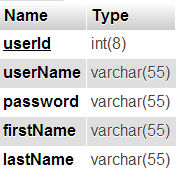# MySQL CONCAT

For MySQL, there are several inbuilt functions, that could be used while writing queries. We have seen some of them, like, MySQL datetime functions, CURDATE(), CURTIME() and etc. Such direct functions will be helpful for performing manipulation with MySQL table data.

We can perform string concatenation by using the direct function provided by MySQL. In MySQL, it contains two functions that could be applied for the purpose of concatenation. These functions are varied from one another, where one of them is having separator while concatenation and the other is not.## MySQL Concatenation Functions

These functions are listed below followed by their detailed description.

• CONCAT() – This will concatenate given a list of string together, continuously, without any separator.
• CONCAT_WS() – Unlike MySQL CONCAT(), this function will join each of the given string, one another, with a separator string.

### CONCAT()

This function simply concatenates given a set of strings that passed as its arguments. And, it is capable of joining two or more number of strings. This function should be used with the following syntax.

```CONCAT(string1,string2,....etc.)
```

Using this syntax, we can expect the result of this function as,

```strin1string2...
```

If we want to add space or anything to separate each string parameter from one another, then, we need to include an additional argument with this function for providing white spaces in between. For example,

```CONCAT(string1,' ',string2)
```

Now, the result will be,

```strin1 string2
```

But, adding a separator in this way will create confusion while adding more number of strings. So, we can use another concatenation function available in MySQL, that’s what next.

### CONCAT_WS()

This function accepts required separator string as its first argument. And, rest of its arguments are the list of strings to be joined together. This function will reduce the burden on adding separator for each pair of subsequent string parameters and makes code clean. The usage of this concatenation function should be,

```CONCAT_WS(<separator-string>,string1,string2);
```

On applying this syntax, we can expect the resultant string returned by this function, as like as,

```strin1<separator-string>string2
```

Note:

• For adding separator string with the result, this function should at least have two string parameter, excluding separator. Rather if we use this function, like,

```CONCAT_WS(<separator-string>,string1);
```

Then, this function will return only string1 without any separator.

• If the separator string is NULL, then will return NULL as its result.

## Applying Concatenation Functions for MySQL Queries

Now, let us see how to apply this concatenation function for MySQL SELECT, INSERT and UPDATE queries.

Insert Query:

```INSERT INTO <table-name> (field1) VALUES (CONCAT(string1,string2...))
```

Update Query:

```UPDATE <table-name> SET field1=CONCAT(string1,string2...)
```

Select Query:

```SELECT CONCAT(string1,string2...) FROM <table-name>
```

Note:

• We should provide strings to be concatenated with MySQL CONCAT(), within quotes.
• Similarly, we can use the CONCAT_WS() function for the queries shown above.

## MySQL CONCAT: Example

Let us have an example PHP program uses query syntax with MySQL CONCAT(). For that, we have taken users table structured as,After creating such table into our database, we need to access from PHP code shown below.

```<?php
\$conn = mysqli_connect("localhost", "root", "test", "blog_samples") or die("Connection Error: " . mysqli_error(\$conn));

\$firstName = "Joel";
\$lastName = "Thomas";
\$rand = rand(1, 1000);

CONCAT('" . \$userName . "','" . \$rand . "'),
'" . \$firstName . "',
'" . \$lastName . "')");
\$current_id = mysqli_insert_id(\$conn);
echo "<b>Row Inserted:</b><br/><br/>";
\$result = mysqli_query(\$conn, "SELECT * FROM users where userId='" . \$current_id . "'");
while (\$row = mysqli_fetch_array(\$result)) {
print "<PRE>";
print_r(\$row);
print "</PRE>";
}

echo "<b>Row Updated:</b><br/><br/>";
\$result = mysqli_query(\$conn, "SELECT * FROM users where userId='" . \$current_id . "'");
while (\$row = mysqli_fetch_array(\$result)) {
print "<PRE>";
print_r(\$row);
print "</PRE>";
}

\$result = mysqli_query(\$conn, "SELECT CONCAT(firstName,lastName) as fullName FROM users where userId='" . \$current_id . "'");
while (\$row = mysqli_fetch_array(\$result)) {
print "<PRE>";
print_r(\$row);
print "</PRE>";
}

\$result = mysqli_query(\$conn, "SELECT CONCAT_WS(' ',firstName,lastName) as fullName FROM users where userId='" . \$current_id . "'");
while (\$row = mysqli_fetch_array(\$result)) {
print "<PRE>";
print_r(\$row);
print "</PRE>";
}
?>
```

After executing this program, the query statement having CONCAT() function reflect expected to change with MySQL users table. And, we can see the effect of each query, by retrieving table content to be displayed to the browser using PHP print statement.